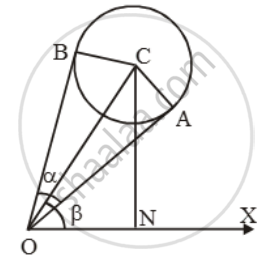# A balloon of radius γ makes an angle α at the eye of an observer and the angle of elevation of its centre is β. Then find the height of its centre from the ground level - Mathematics

Sum

A balloon of radius γ makes an angle α at the eye of an observer and the angle of elevation of its centre is β. Then find the height of its centre from the ground level

#### Solution

Let C be the centre of the balloon and O be the position of the observer at the horizontal line OX.

Let OA and OB be the tangents to the balloon so that ∠AOB = α, ∠XOC = β and

CA = CB = γ.Clearly, right angled triangles OAC and OBC are congruent.

∠AOC = ∠BOC = α/2.

Let CN ⊥ OX.

\text{Now, }\frac{OC}{CA}=\text{cosec }\frac{\alpha }{2}

\Rightarrow OC=\gamma \text{ cosec }\frac{\alpha}{2}\text{ }...\text{(i)}

\text{Also, }\frac{CN}{OC}=\sin \beta

\Rightarrow CN=OC\text{ sin }\beta =\gamma \text{ cosec }\frac{\alpha }{2}\sin \beta \

Concept: Heights and Distances
Is there an error in this question or solution?